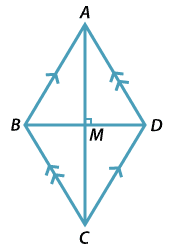### Using congruence to establish a test for rhombuses

##### Theorem

A quadrilateral whose diagonals bisect each other at right angles is a rhombus.

##### ProofLet $$ABCD$$ be a quadrilateral whose diagonals bisect each other at right angles at M.

We prove that $$DA = AB$$. It follows similarly that

$$AB = BC\ \text{and} \ BC = CD$$

$$\triangle ABC \equiv \triangle CDA$$ (AAS)

So $$AB = AD$$ and by the first test above $$ABCD$$ is a rhombus.